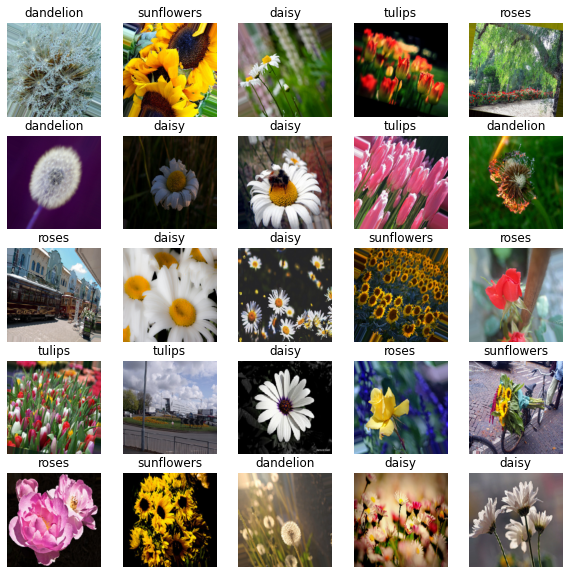Data augmentation is a favorite recipe among deep learning practitioners especially for the ones working in the field of computer vision. Data augmentation is a technique used for introducing variety in training data thereby helping to mitigate overfitting.

When using Keras for training image classification models, using the ImageDataGenerator class for handling data augmentation is pretty much a standard choice. However, with TensorFlow, we get a number of different ways we can apply data augmentation to image datasets. In this tutorial, we are going to discuss three such ways. Knowing about these different ways of plugging in data augmentation in your image classification training pipelines will help you decide the best way for a given scenario.

Here’s a brief overview of the different ways we are going to cover:

• Using the standard ImageDataGenerator class
• Using TensorFlow image ops with a TensorFlow dataset
• Using Keras’s (experimental) image processing layers
• Mix-matching different image ops & image processing layers

Let’s get started!

# Experimental setup

We are going to use the flowers dataset to demonstrate the experiments. Downloading the dataset is just as easy as executing the following line of code:

flowers contains the path (which in my case is - /root/.keras/datasets/flower_photos) where the dataset got downloaded. The structure of the dataset looks like so -

├── daisy [633 entries]
├── dandelion 
├── roses 
├── sunflowers [699 entries]
├── tulips [799 entries]
└── LICENSE.txt
# Get the flowers dataset
flowers = tf.keras.utils.get_file(
'flower_photos',
untar=True)


Using the standard ImageDataGenerator class For most of the scenarios, the ImageDataGenerator should be good enough. Its flexible API design is really to follow and it makes it easier to work with custom image datasets by providing meaningful high-level abstractions.

We instantiate the ImageDataGenerator class like so -

img_gen = tf.keras.preprocessing.image.ImageDataGenerator(
rescale=1./255,
rotation_range=30,
horizontal_flip=True)


We specify two augmentation operations and a pixel rescaling operation in there. ImageDataGenerator comes with a handy flow_from_directory method that allows us to read images from a directory and apply the specified operations on the fly during the time of training. Here’s how to instruct the img_gen object to read images from a directory -

IMG_SHAPE = 224
BATCH_SIZE = 32

img_flow = img_gen.flow_from_directory(flowers,
shuffle=True,
batch_size=BATCH_SIZE,
target_size=(IMG_SHAPE, IMG_SHAPE))

Found 3670 images belonging to 5 classes.


We then verify the images and the labels and they are indeed parsed right -

images, labels = next(img_flow)
print(images.shape, labels.shape)
show_batch(images, labels)

(32, 224, 224, 3) (32, 5)Training with an ImageDataGenerator instance is extremely straight-forward -

model = get_training_model()
model.fit(img_flow, ...)


For a fully worked out example, refer to this tutorial.

As can be seen in this blog post, ImageDataGenerator’s overall data loading performance can have a significant effect on how fast your model trains. To tackle situations, where you need to maximize the hardware utilization without burning unnecessary bucks, TensorFlow’s data module can be really helpful (comes at some costs).

# TensorFlow image ops with tf.data APIs

The blog post I mentioned in the previous section shows the kind of performance boost achievable with tf.data APIs. But it’s important to note that boost comes at the cost of writing boilerplate code which makes the overall process more involved. For example, here’s how you would load and preprocess your images and labels -

def parse_images(image_path):
# Load and preprocess the image
img = tf.image.decode_jpeg(img, channels=3) # decode the image back to proper format
img = tf.image.convert_image_dtype(img, tf.float32) # scale the pixel values to [0, 1]
img = tf.image.resize(img, [IMG_SHAPE, IMG_SHAPE]) # resize the image

# Parse the labels
label = tf.strings.split(image_path, os.path.sep)

return (img, label)


You would then write a separate augmentation policy with the TensorFlow Image ops -

def augment(image, label):
img = tf.image.rot90(image)
img = tf.image.flip_left_right(img)
return (img, label)


To chain the above two together you would first create an initial dataset consisting of only the image paths -

image_paths = list(paths.list_images(flowers))
list_ds = tf.data.Dataset.from_tensor_slices((image_paths))


Now, you would read, preprocess, shuffle, augment, and batch your dataset -

AUTO = tf.data.experimental.AUTOTUNE

train_ds = (
list_ds
.map(parse_images, num_parallel_calls=AUTO)
.shuffle(1024)
.map(augment, num_parallel_calls=AUTO) # augmentation call
.batch(BATCH_SIZE)
.prefetch(AUTO)
)


num_parallel_calls allows you to parallelize the mapping function and tf.data.experimental.AUTOTUNE lets TensorFlow decide the level of parallelism to use dynamically (how cool is that?). prefetch allows loading in the next batch of data well before your model finishes the current epoch of training. It is evident that this process is more involved than the previous one.

Verifying if we constructed the data input pipeline correctly is a vital step before you feed your data to the model -

image_batch, label_batch = next(iter(train_ds))
print(image_batch.shape, label_batch.shape)
show_batch(image_batch.numpy(), label_batch.numpy(), image_data_gen=False)

(32, 224, 224, 3) (32,)